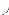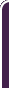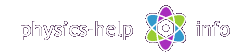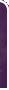homephysics online guidephysics help my info terms of use contactsWhat is Physics? Mechanics Electricity and Magnetism Electric Field Gauss' Law about below subjects Electric Potential Capacity Direct Current Magnetic Field Magnetic Field Laws Magnetic Interactions Electromagnetic Induction Maxwell's Equations Oscillations and Waves Simple Harmonic Motion Damped Harmonic Motion Driven Harmonic Motion Electric Oscillation Alternating Current Wave Motion Elastic Waves Electromagnetic Waves Optics Light Waves Geometrical Optics Interference Polarization Diffraction Fraunhofer Diffraction Dispersion, Absorption, Diffusion Doppler Effect Thermodynamics Ideal Gas Molecular Statistics Transport Phenomena First Law of Thermodynamics Second and Third Laws of Thermodynamics Imperfect Gas Liquids Solids Quantum Physics Thermal Radiation Quantum Properties of Light Wave Properties of Particles Planetary Model of Atom X-Rays Particle in Potential Well Pauli Exclusion Principle Nuclear Physics Solid State Physics Appendices# Gauss' Law in Electrostatics   short version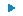SI units & Physics constants

Electrostatics investigates interaction between fixed electric charges

The Gauss' Law is used to find electric field when the charge is continuously distributed within an object with symmetrical geometry, such as sphere, cylinder, or plane. Gauss' law follows Coulomb's law and the Superposition Principle.

## Electric flux

Consider electric fieldand choose mentally a plane surface of area A with normal unit vector(with magnitude u = 1), oriented at anglewith respect to the field as shown belowLet the area be so small that the electric field is uniform everywhere on it.

The electric flux thorough the area A is defined as scalar productThe above definition defines the units of electric flux as volt times meterNow we can define the electric flux through any surface in an arbitrary electric field. We should subdivide this surface into n small elements each of area, and find the electric fluxes through all elements using the above formula, forwhere:is the electric field at the center of;is  the angle betweenand normal to the plane of the element.

Since electric flux is a scalar value, the total electric flux through the surface is given by the sumHere the smaller valuethe more n, and the more accurate value of the electric flux is given.

Two important cases follows from the above formula:

1. When the electric field everywhere is parallel to the surface the electric flux through the surface is zero, since hereand.

2. When the electric field is perpendicular everywhere to the surface the electric flux through the surface is given byIn particular, if the electric field everywhere is normal to the surface and has the same value E, then the formula for the electric flux is:where A is the total area of the surface

Using the electric field lines concept, let us find the obvious physical meaning of the electric flux. Puttingandin formula for electric flux, we get the number of electric field lines crossing the element of areaNow putting it into electric flux, we getThus the net electric flux through any surface is equal numerically to the total number of electric lines, N, crossing the surface.

Now consider the general case when the electric fieldis produced by a system of point charges Qi for i = 1,2,3,...n. Draw mentally a closed surface with area A around the charges so they all are enclosed within the surface. Such surface is called Gauss' surface.According to formula for total number of electric field lines, each charge produces number of electric lanes crossing the surface A given byforUsing formula for electric flux we get that the total electric flux through the surface isNow the left and right parts in the above equation have the same units, andis the net charge enclosed within the surface. So we get the equalityThis equation is called Gauss' law. Thus Gauss' law states that electric flux through any closed surface is equal to the net charge enclosed inside the surface divided by permittivity of vacuum. The reader can find more rigorous proof of Gauss' law in any high level Physics book.

Gauss' law is valid for any discrete set of point charges. Nevertheless, this law is also valid when an electric field is produced by charged objects with continuously distributed charges, since any continuously distributed charge is a combination of elementary point charges.

Gauss' law usually is used to calculate the electric field produced by an object charged with symmetrical charge distribution. As an example, find the electric field outside the charged sphere. Consider the case when the electric field is produced by the sphere of radius R symmetrically charged by charge QThe first step of using Gauss' law is always the consideration of symmetry. Since the electric field is produced by charges, the symmetry of the field will repeat the symmetry of the charges. So the electric field of a spherically symmetrical charged sphere will also have the spherical symmetry. The electric field is directed along the radius from the center of the sphere with a magnitude defined by the magnitude of the radius. Therefore let us take Gauss' surface, A, as a sphere of radiusand areaconcentric with the charged sphere as shown above. Now the electric field on the Gauss' sphere is normal to the surface and has the same magnitude. So according to the above formula for case 2 the net flux through the sphere is given byOn the other hand, Gauss' law showsEquating these two equations givesFrom which we get the magnitude of the electric field outside the charged sphere at a distance r from its centerThis is the exact same formula as the formula for electric field which was found earlier, using Coulomb's law for electric field of point charge Q located at the center of the sphere. The important result is that Coulomb's law formulated initially for point charges can be used as well for charged spheres.

Exercise: Show, using Gauss' law, that the electric field inside the sphere of radius R, uniformly charged by charge Q, atis given byHint: Take Gauss’ surface as a sphere of radius r<R and find the enclosed charge

Consider the next very important case when the electric field is produced by an infinite plane, charged uniformly by a surface charge density. The charge density is defined as the charge per unit of the plane areaHere we have axial symmetry in the direction normal to the plane, and so the electric field is directed everywhere from the plane in the normal direction as shown in the above diagram (for a positively charged plane).

Using this symmetry, insert Gauss’ surface as a closed cylinder with cross section A. The electric field everywhere on the lateral surface is parallel to the surface, and so according to case 1 the electric flux through the lateral surface is zero. On the faceplates the electric field is normal to the surface and has a constant value E, so according to case 2 the net electric flux through the cylinder is given byAccording to the definition of surface charge density, the charge enclosed within the cylinder is given byPuttingand Q into Gauss' Law givesFrom which we get the magnitude of the electric field produced by the planeWe got the important result, that an electric field near any charged plane is uniform everywhere, if the distance from the plane is small compared with the dimensions of the plane.

Exercise: Show, using Gauss' law, that the electric field produced by an infinitely long straight line, uniformly charged by a linear charge density, at a distance r from the line is directed everywhere perpendicular to the line and has a magnitude given byHint: Take Gauss’ surface as a cylinder of radius r with axis on the line and find the enclosed charge, using cylindrical symmetry

## Conductors in an electric field

Regarding the capability of charges to move inside a substance, all substances are subdivided into two large groups: dielectrics and conductors. In dielectrics all negative and positive charges are fixed and cannot move inside the substance under the influence of usual external forces. Contrary to dielectrics, in conductors, the electrons of the outer valence shells are collectivized by all atoms of the substance due to interatomic interaction. As a result these electrons are not bounded by atoms and can freely move through entire substance under the influence of negligibly small electric forces.

Consider a conductor at equilibrium placed into external electrostatic field, with free charge q inside of itOne readily can see that at equilibrium all free charges will be rearranged so that the net internal electric forceon the charge q is zero. Otherwise this charge will move inside the conductor, until it finds the place where. The net electric field inside the conductor is given byThus we get, that at equilibrium the internal electric field inside the conductor is always zero.

To understand how the free electrons are distributed in a conductor let us use the Gauss' law. Consider a conductor with its outer surface A charged by charge Q. Draw two Gauss’ surfaces A1 and A2 near the surface A separated by infinitely thin layer as shown below, where A1 is drawn inside the conductor and A2 outside the conductor.The electric field inside the conductor is zero. So the electric flux through A1 is zero, and according to Gauss' law the electric charge inside A1 must be zero. On the other hand for A2 the enclosed charge is equal to the total charge Q. Since A1 and A2 are infinitely close to each other, we conclude that the charge Q can be located only on the surface of the conductor distributed with a surface charge density.

Find the direction of the electric fieldon the surface of the conductor. We can readily understand that at equilibrium the tangent component Et of the electric field must be zero. Otherwise the flow of free electrons of the conductor moves over the surface under the tangent electric force produced by Et and the surface charge will be rearranged so that Et = 0.Thus the electric field on the surface of a conductor is always directed normal to the surface.

The value of this field can be found from Gauss' law. Consider a conductor charged by the surface charge densityRepeat the procedure that we used above for the electric field from the charged plane. Draw a small right cylinder whose end faces A1 and A2 and lateral surface A3 with the axis along the electric field as shown in the above diagram. The cylinder must be small enough so that the part of the surface of the conductor inside the cylinder can be considered as a flat plane. Now the electric field inside the conductor is zero, and the electric field outside the conductor is normal to the plane. So the net electric flux through the cylinder is given byThe net charge enclosed by cylinder is given byPuttingand Q into Gauss' Law givesFrom which we get the magnitude of the electric field on the surface of the conductorWith this information, it is now known what happens when an uncharged conductor is inserted into an external electric field. According to the above consideration the electric field lines near the surface of the conductor are distorted, so that they become normal to the surface as shown below. As a result, according to the above formula, the electric field induces the charges equal in magnitude and opposite in direction on the opposite faces of the conductor . This phenomenon is called electrostatic polarization of a conductorhome  |  physics online guide  |  physics help  |  my info  |  terms of use  |  contacts Dr. Nikitin © 2010